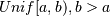# numpy.random.ranf¶

`numpy.random.``ranf`(size=None)

Return random floats in the half-open interval [0.0, 1.0).

Results are from the “continuous uniform” distribution over the stated interval. To samplemultiply the output of `random_sample` by (b-a) and add a:

```(b - a) * random_sample() + a
```
Parameters: size : int or tuple of ints, optional Output shape. If the given shape is, e.g., `(m, n, k)`, then `m * n * k` samples are drawn. Default is None, in which case a single value is returned. out : float or ndarray of floats Array of random floats of shape size (unless `size=None`, in which case a single float is returned).

Examples

```>>> np.random.random_sample()
0.47108547995356098
>>> type(np.random.random_sample())
<type 'float'>
>>> np.random.random_sample((5,))
array([ 0.30220482,  0.86820401,  0.1654503 ,  0.11659149,  0.54323428])
```

Three-by-two array of random numbers from [-5, 0):

```>>> 5 * np.random.random_sample((3, 2)) - 5
array([[-3.99149989, -0.52338984],
[-2.99091858, -0.79479508],
[-1.23204345, -1.75224494]])
```

#### Previous topic

numpy.random.random

#### Next topic

numpy.random.sample